# summary

Generate summary table of backtest results

Since R2020b

## Syntax

``summaryTable = summary(backtester)``

## Description

example

````summaryTable = summary(backtester)` generates a table of metrics to summarize the backtest. Each row of the table is a calculated metric and each column represents a strategy. You must run the summary function only after running the `runBacktest` function.```

## Examples

collapse all

The MATLAB® backtesting engine runs backtests of portfolio investment strategies over time series of asset price data. You can use `summary` to compare multiple strategies over the same market scenario. This example shows how to examine the results of a backtest with two strategies.

Load one year of stock price data. For readability, this example uses a subset of the DJIA stocks.

```% Read table of daily adjusted close prices for 2006 DJIA stocks T = readtable('dowPortfolio.xlsx'); % Prune the table to include only the dates and selected stocks timeColumn = "Dates"; assetSymbols = ["BA", "CAT", "DIS", "GE", "IBM", "MCD", "MSFT"]; T = T(:,[timeColumn assetSymbols]); % Convert to timetable pricesTT = table2timetable(T,'RowTimes','Dates'); % View the final asset price timetable head(pricesTT)```
``` Dates BA CAT DIS GE IBM MCD MSFT ___________ _____ _____ _____ _____ _____ _____ _____ 03-Jan-2006 68.63 55.86 24.18 33.6 80.13 32.72 26.19 04-Jan-2006 69.34 57.29 23.77 33.56 80.03 33.01 26.32 05-Jan-2006 68.53 57.29 24.19 33.47 80.56 33.05 26.34 06-Jan-2006 67.57 58.43 24.52 33.7 82.96 33.25 26.26 09-Jan-2006 67.01 59.49 24.78 33.61 81.76 33.88 26.21 10-Jan-2006 67.33 59.25 25.09 33.43 82.1 33.91 26.35 11-Jan-2006 68.3 59.28 25.33 33.66 82.19 34.5 26.63 12-Jan-2006 67.9 60.13 25.41 33.25 81.61 33.96 26.48 ```

The inverse variance strategy requires some price history to initialize, so you can allocate a portion of the data to use for setting initial weights. By doing this, you can "warm start" the backtest.

```warmupRange = 1:20; testRange = 21:height(pricesTT);```

Create Strategies

Define an investment strategy by using the `backtestStrategy` function. This example builds two strategies:

• Equal weighted

• Inverse variance

This example does not provide details on how to build the strategies. For more information on creating strategies, see `backtestStrategy`. The strategy rebalance functions are implemented in the Rebalance Functions section.

```% Create the strategies ewInitialWeights = equalWeightFcn([],pricesTT(warmupRange,:)); ewStrategy = backtestStrategy("EqualWeighted",@equalWeightFcn, ... 'RebalanceFrequency',20, ... 'TransactionCosts',[0.0025 0.005], ... 'LookbackWindow',0, ... 'InitialWeights',ewInitialWeights)```
```ewStrategy = backtestStrategy with properties: Name: "EqualWeighted" RebalanceFcn: @equalWeightFcn RebalanceFrequency: 20 TransactionCosts: [0.0025 0.0050] LookbackWindow: 0 InitialWeights: [0.1429 0.1429 0.1429 0.1429 0.1429 0.1429 0.1429] ManagementFee: 0 ManagementFeeSchedule: 1y PerformanceFee: 0 PerformanceFeeSchedule: 1y PerformanceHurdle: 0 UserData: [0x0 struct] EngineDataList: [0x0 string] ```
```ivInitialWeights = inverseVarianceFcn([],pricesTT(warmupRange,:)); ivStrategy = backtestStrategy("InverseVariance",@inverseVarianceFcn, ... 'RebalanceFrequency',20, ... 'TransactionCosts',[0.0025 0.005], ... 'InitialWeights',ivInitialWeights)```
```ivStrategy = backtestStrategy with properties: Name: "InverseVariance" RebalanceFcn: @inverseVarianceFcn RebalanceFrequency: 20 TransactionCosts: [0.0025 0.0050] LookbackWindow: [0 Inf] InitialWeights: [0.1401 0.0682 0.0795 0.2187 0.1900 0.1875 0.1160] ManagementFee: 0 ManagementFeeSchedule: 1y PerformanceFee: 0 PerformanceFeeSchedule: 1y PerformanceHurdle: 0 UserData: [0x0 struct] EngineDataList: [0x0 string] ```
```% Aggregate the strategies into an array strategies = [ewStrategy ivStrategy];```

Run Backtest

Create a backtesting engine and run a backtest over a year of stock data. For more information on creating backtesting engines, see `backtestEngine`. The software initializes several properties of the `backtestEngine` object to empty. These read-only properties are populated by the engine after you run the backtest.

```% Create the backtesting engine using the default settings backtester = backtestEngine(strategies)```
```backtester = backtestEngine with properties: Strategies: [1x2 backtestStrategy] RiskFreeRate: 0 CashBorrowRate: 0 RatesConvention: "Annualized" Basis: 0 InitialPortfolioValue: 10000 DateAdjustment: "Previous" PayExpensesFromCash: 0 NumAssets: [] Returns: [] Positions: [] Turnover: [] BuyCost: [] SellCost: [] TransactionCosts: [] Fees: [] ```

Run the backtest using `runBacktest`.

```% Run the backtest backtester = runBacktest(backtester,pricesTT(testRange,:));```

Examine Summary Results

The `summary` function uses the results of the backtest and returns a table of high-level results from the backtest.

`s1 = summary(backtester)`
```s1=9×2 table EqualWeighted InverseVariance _____________ _______________ TotalReturn 0.17567 0.17155 SharpeRatio 0.097946 0.10213 Volatility 0.0074876 0.0069961 AverageTurnover 0.0007014 0.0024246 MaxTurnover 0.021107 0.097472 AverageReturn 0.00073178 0.00071296 MaxDrawdown 0.097647 0.096299 AverageBuyCost 0.018532 0.061913 AverageSellCost 0.037064 0.12383 ```

Each row of the table output is a measurement of the performance of a strategy. Each strategy occupies a column. The `summary` function reports on the following metrics:

• `TotalReturn` — The nonannulaized total return of the strategy, inclusive of fees, over the full backtest period.

• `SharpeRatio `— The nonannualized Sharpe ratio of each strategy over the backtest. For more information, see `sharpe`.

• `Volatility `— The nonannualized standard deviation of per-time-step strategy returns.

• `AverageTurnover `— The average per-time-step portfolio turnover, expressed as a decimal percentage.

• `MaxTurnover `— The maximum portfolio turnover in a single rebalance, expressed as a decimal percentage.

• `AverageReturn` —The arithmetic mean of the per-time step portfolio returns.

• `MaxDrawdown `— The maximum drawdown of the portfolio, expressed as a decimal percentage. For more information, see `maxdrawdown`.

• `AverageBuyCost `— The average per-time-step transaction costs the portfolio incurred for asset purchases.

• `AverageSellCost `— The average per-time-step transaction costs the portfolio incurred for asset sales.

Sometimes it is useful to transpose the `summary` table when plotting the metrics of different strategies.

```s2 = rows2vars(s1); s2.Properties.VariableNames{1} = 'StrategyName'```
```s2=2×10 table StrategyName TotalReturn SharpeRatio Volatility AverageTurnover MaxTurnover AverageReturn MaxDrawdown AverageBuyCost AverageSellCost ___________________ ___________ ___________ __________ _______________ ___________ _____________ ___________ ______________ _______________ {'EqualWeighted' } 0.17567 0.097946 0.0074876 0.0007014 0.021107 0.00073178 0.097647 0.018532 0.037064 {'InverseVariance'} 0.17155 0.10213 0.0069961 0.0024246 0.097472 0.00071296 0.096299 0.061913 0.12383 ```
```bar(s2.AverageTurnover) title('Average Turnover') ylabel('Average Turnover (%)') set(gca,'xticklabel',s2.StrategyName)```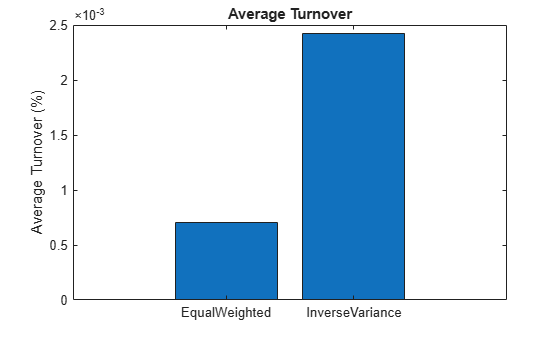Examine Detailed Results

After you run the backtest, the `backtestEngine` object updates the read-only fields with the detailed results of the backtest. The `Returns`, `Positions`, `Turnover`, `BuyCost`, `SellCost`, and `Fees` properties each contain a timetable of results. Since this example uses daily price data in the backtest, these timetables hold daily results.

`backtester`
```backtester = backtestEngine with properties: Strategies: [1x2 backtestStrategy] RiskFreeRate: 0 CashBorrowRate: 0 RatesConvention: "Annualized" Basis: 0 InitialPortfolioValue: 10000 DateAdjustment: "Previous" PayExpensesFromCash: 0 NumAssets: 7 Returns: [230x2 timetable] Positions: [1x1 struct] Turnover: [230x2 timetable] BuyCost: [230x2 timetable] SellCost: [230x2 timetable] TransactionCosts: [1x1 struct] Fees: [1x1 struct] ```

Returns

The `Returns` property holds a timetable of strategy (simple) returns for each time step. These returns are inclusive of all transaction fees.

`head(backtester.Returns)`
``` Time EqualWeighted InverseVariance ___________ _____________ _______________ 02-Feb-2006 -0.007553 -0.0070957 03-Feb-2006 -0.0037771 -0.003327 06-Feb-2006 -0.0010094 -0.0014312 07-Feb-2006 0.0053284 0.0020578 08-Feb-2006 0.0099755 0.0095781 09-Feb-2006 -0.0026871 -0.0014999 10-Feb-2006 0.0048374 0.0059589 13-Feb-2006 -0.0056868 -0.0051232 ```
```binedges = -0.025:0.0025:0.025; h1 = histogram(backtester.Returns.EqualWeighted,'BinEdges',binedges); hold on histogram(backtester.Returns.InverseVariance,'BinEdges',binedges); hold off title('Distribution of Daily Returns') legend([strategies.Name]);```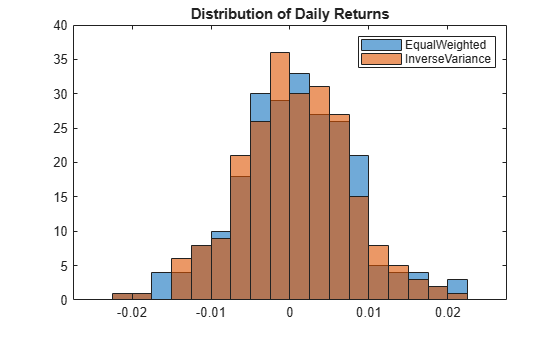Positions

The `Positions` property holds a structure of timetables, one per strategy.

`backtester.Positions`
```ans = struct with fields: EqualWeighted: [231x8 timetable] InverseVariance: [231x8 timetable] ```

The `Positions` timetable of each strategy holds the per-time-step positions for each asset as well as the `Cash` asset (which earns the risk-free rate). The `Positions` timetables contain one more row than the other results timetables because the `Positions` timetables include initial positions of the strategy as their first row. You can consider the initial positions as the `Time` = `0` portfolio positions. In this example, the `Positions` timetables start with February 1, while the others start on February 2.

`head(backtester.Positions.InverseVariance)`
``` Time Cash BA CAT DIS GE IBM MCD MSFT ___________ ___________ ______ ______ ______ ______ ______ ______ ______ 01-Feb-2006 0 1401.2 682.17 795.14 2186.8 1900.1 1874.9 1159.8 02-Feb-2006 0 1402.8 673.74 789.74 2170.8 1883.5 1863.6 1145 03-Feb-2006 1.0987e-12 1386.5 671.2 787.2 2167.3 1854.3 1890.5 1139 06-Feb-2006 1.0971e-12 1391.9 676.78 785.62 2161.1 1843.6 1899.1 1123.8 07-Feb-2006 0 1400 661.66 840.23 2131.9 1851.6 1902.3 1114.5 08-Feb-2006 -2.2198e-12 1409.8 677.9 846.58 2160.4 1878.2 1911 1113.2 09-Feb-2006 0 1414.8 674.35 840.87 2172.2 1869 1908.3 1102.6 10-Feb-2006 0 1425.1 677.29 839.6 2195.8 1890.6 1909.3 1103.9 ```
```% Plot the change of asset allocation over time t = backtester.Positions.InverseVariance.Time; positions = backtester.Positions.InverseVariance.Variables; h = area(t,positions); title('Inverse Variance Positions'); xlabel('Date'); ylabel('Asset Positions'); datetick('x','mm/dd','keepticks'); ylim([0 12000]) xlim([t(1) t(end)]) cm = parula(numel(h)); for i = 1:numel(h) set(h(i),'FaceColor',cm(i,:)); end```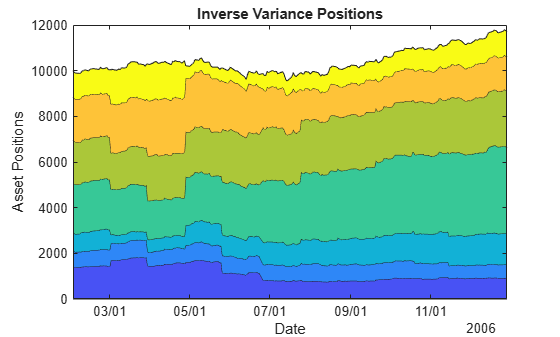Turnover

The `Turnover` timetable holds the per-time-step portfolio turnover.

`head(backtester.Turnover)`
``` Time EqualWeighted InverseVariance ___________ _____________ _______________ 02-Feb-2006 0 0 03-Feb-2006 0 0 06-Feb-2006 0 0 07-Feb-2006 0 0 08-Feb-2006 0 0 09-Feb-2006 0 0 10-Feb-2006 0 0 13-Feb-2006 0 0 ```

Depending on your rebalance frequency, the `Turnover` table can contain mostly zeros. Removing these zeros when you visualize the portfolio turnover is useful.

```nonZeroIdx = sum(backtester.Turnover.Variables,2) > 0; to = backtester.Turnover(nonZeroIdx,:); plot(to.Time,to.EqualWeighted,'-o',to.Time,to.InverseVariance,'-x',... 'LineWidth',2,'MarkerSize',5); legend([strategies.Name]); title('Portfolio Turnover'); ylabel('Turnover (%)');```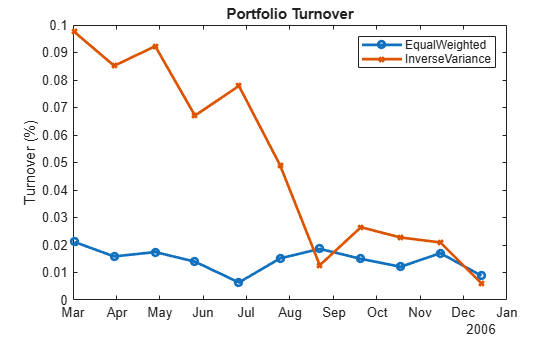The `BuyCost` and `SellCost` timetables hold the per-time-step transaction fees for each type of transaction, purchases, and sales.

```totalCost = sum(backtester.BuyCost{:,:}) + sum(backtester.SellCost{:,:}); bar(totalCost); title('Total Transaction Costs'); ylabel('\$') set(gca,'xticklabel',[strategies.Name])```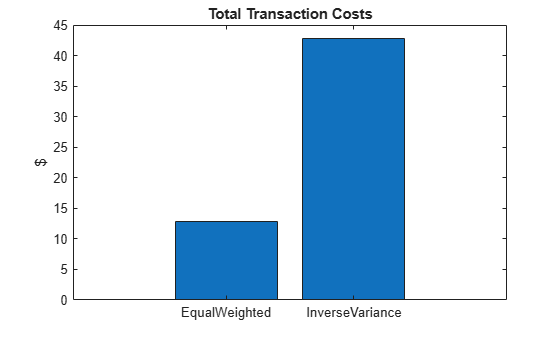Generate Equity Curve

Use `equityCurve` to plot the equity curve for the equal weighted and inverse variance strategies.

`equityCurve(backtester)`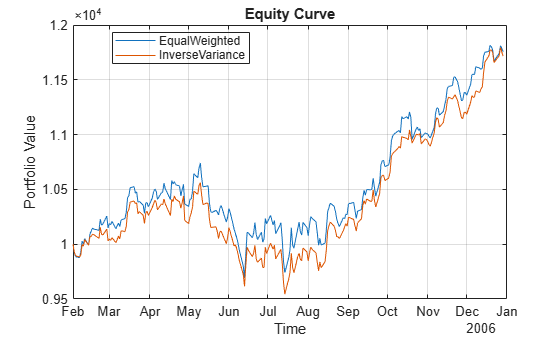Rebalance Functions

This section contains the implementation of the strategy rebalance functions. For more information on creating strategies and writing rebalance functions, see `backtestStrategy`.

```function new_weights = equalWeightFcn(current_weights, pricesTT) %#ok<INUSL> % Equal weighted portfolio allocation nAssets = size(pricesTT, 2); new_weights = ones(1,nAssets); new_weights = new_weights / sum(new_weights); end```
```function new_weights = inverseVarianceFcn(current_weights, pricesTT) %#ok<INUSL> % Inverse-variance portfolio allocation assetReturns = tick2ret(pricesTT); assetCov = cov(assetReturns{:,:}); new_weights = 1 ./ diag(assetCov); new_weights = new_weights / sum(new_weights); end```

## Input Arguments

collapse all

Backtesting engine, specified as a `backtestEngine` object. Use `backtestEngine` to create the backtesting engine and then use `runBacktest` to run a backtest.

Data Types: `object`

## Output Arguments

collapse all

Metrics summarizing the backtest, returned as a table where each row of the table is a calculated metric and each column represents a strategy. The reported metrics are as follows:

• `TotalReturn` — The total return of the strategy over the entire backtest

• `SharpeRatio` — The Sharpe ratio for each strategy

• `Volatility` — The volatility of each strategy over the backtest

• `AverageTurnover` — Average turnover per-time-step as a decimal percent

• `MaxTurnover` — Maximum turnover in a single time step

• `AverageReturn` — Average return per-time-step

• `MaxDrawdown` — Maximum portfolio drawdown as a decimal percent

• `AverageBuyCost` — Average per-time-step transaction costs for asset purchases

• `AverageSellCost` — Average per-time-step transaction costs for asset sales

## Version History

Introduced in R2020b